# Python | Pandas dataframe.infer_objects()

• Last Updated : 19 Nov, 2018

Python is a great language for doing data analysis, primarily because of the fantastic ecosystem of data-centric python packages. Pandas is one of those packages and makes importing and analyzing data much easier.

Pandas` dataframe.infer_objects()` function attempts to infer better data type for input object column. This function attempts soft conversion of object-dtyped columns, leaving non-object and unconvertible columns unchanged. The inference rules are the same as during normal Series/DataFrame construction.

Syntax: DataFrame.infer_objects()
Returns : converted : same type as input object

Example #1: Use `infer_objects()` function to infer better data type.

 `# importing pandas as pd``import` `pandas as pd`` ` `# Creating the dataframe ``df ``=` `pd.DataFrame({``"A"``:[``"sofia"``, ``5``, ``8``, ``11``, ``100``],``                   ``"B"``:[``2``, ``8``, ``77``, ``4``, ``11``],``                   ``"C"``:[``"amy"``, ``11``, ``4``, ``6``, ``9``]})`` ` `# Print the dataframe``df`

Output :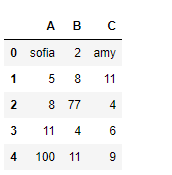Let’s see the dtype (data type) of each column in the dataframe.

 `# to print the basic info``df.info()`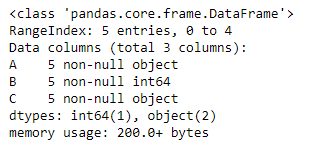As we can see in the output, first and third column is of `object` type. whereas the second column is of `int64` type. Now slice the dataframe and create a new dataframe from it.

 `# slice from the 1st row till end``df_new ``=` `df[``1``:]`` ` `# Let's print the new data frame``df_new`` ` `# Now let's print the data type of the columns``df_new.info()`

Output :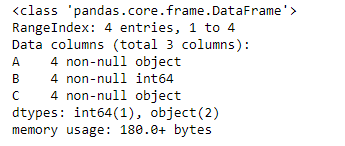As we can see in the output, column “A” and “C” are of object type even though they contain integer value. So, let’s try the `infer_objects()` function.

 `# applying infer_objects() function.``df_new ``=` `df_new.infer_objects()`` ` `# Print the dtype after applying the function``df_new.info()`

Output :Now, if we look at the dtype of each column, we can see that the column “A” and “C” are now of `int64` type.

Example #2: Use `infer_objects()` function to infer better data type for the object.

 `# importing pandas as pd``import` `pandas as pd`` ` `# Creating the dataframe ``df ``=` `pd.DataFrame({``"A"``:[``"sofia"``, ``5``, ``8``, ``11``, ``100``], ``                   ``"B"``:[``2` `+` `2j``, ``8``, ``77``, ``4``, ``11``],``                   ``"C"``:[``"amy"``, ``11``, ``4``, ``6``, ``9``]})`` ` `# Print the dataframe``df`Let’s see the dtype (data type) of each column in the dataframe.

 `# to print the basic info``df.info()`As we can see in the output, first and third column is of `object` type. whereas the second column is of `complex128` type. Now slice the dataframe and create a new dataframe from it.

 `# slice from the 1st row till end``df_new ``=` `df[``1``:]`` ` `# Let's print the new data frame``df_new`` ` `# Now let's print the data type of the columns``df_new.info()`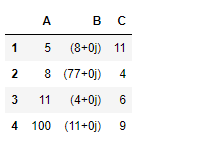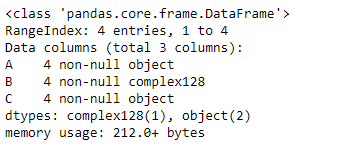As we can see in the output, column “A” and “C” are of object type even though they contain integer value. Similar is the case with column “B”. So, let’s try the `infer_objects()` function.

 `# applying infer_objects() function.``df_new ``=` `df_new.infer_objects()`` ` `# Print the dtype after applying the function``df_new.info()`

Output :Notice, the dtype for column “B” did not change. `infer_objects()` function tries to do soft conversion leaving non-object and unconvertible columns unchanged.

My Personal Notes arrow_drop_up